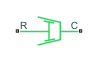# Nonlinear Rotational Damper

Nonlinear damper in a rotational system

•Libraries:
Simscape / Driveline / Couplings & Drives / Springs & Dampers

## Description

The Nonlinear Rotational Damper block represents a nonlinear rotational damper. Polynomial and table lookup parameterizations define the nonlinear relationship between damping torque and relative angular velocity. The damping torque can be symmetric or asymmetric about the zero velocity point. The block applies equal and opposite damping torques on the two rotational conserving ports.

The symmetric polynomial parameterization defines the damping torque for both positive and negative relative velocities according to the expression:

`$T={b}_{1}\omega +\mathrm{sign}\left(\omega \right)\cdot {b}_{2}{\omega }^{2}+{b}_{3}{\omega }^{3}+\mathrm{sign}\left(\omega \right)\cdot {b}_{4}{\omega }^{4}+{b}_{5}{\omega }^{5},$`

where:

• T — Damping torque

• b1, b2, ..., b5 — Damping coefficients

• ω — Relative angular velocity between ports R and C, $\omega ={\omega }_{R}-{\omega }_{C}$

• ωR — Absolute angular velocity associated with port R

• ωC — Absolute angular velocity associated with port C

To avoid zero-crossings that slow simulation, eliminate the sign function from the polynomial expression by specifying an odd polynomial (b2,b4 = 0).

The two-sided polynomial parameterization defines the damping torque for both positive and negative relative velocities according to the expression:

`$T=\left\{\begin{array}{cc}{b}_{1p}\omega +{b}_{2p}{\omega }^{2}+{b}_{3p}{\omega }^{3}+{b}_{4p}{\omega }^{4}+{b}_{5p}{\omega }^{5},& \omega \ge 0\\ {b}_{1n}\omega +{b}_{2n}{\omega }^{2}+{b}_{3n}{\omega }^{3}+{b}_{4n}{\omega }^{4}+{b}_{5n}{\omega }^{5},& \omega <0\end{array},$`

where:

• b1p, b2p, ..., b5p — Damping coefficients for positive relative velocities

• b1n, b2n, ..., b5n — Damping coefficients for negative relative velocities

Both polynomial parameterizations use a fifth-order polynomial expression. To use a lower-order polynomial, set the unneeded higher-order coefficients to zero. To use a higher-order polynomial, fit to a lower-order polynomial or use the table lookup parameterization.

The table lookup parameterization defines damping torque based on a set of torque and angular velocity vectors. If not specified, the block automatically adds a data point at the origin, that is, the intersection of zero angular velocity and zero torque.

### Assumptions and Limitations

The block assumes viscous damping. The damping force depends only on velocity.

## Ports

### Conserving

expand all

Mechanical translational conserving port associated with the shaft.

Mechanical translational conserving port associated with the case.

## Parameters

expand all

Whether to parameterize damping by polynomial or table lookup.

Whether to use a symmetric or two-sided table lookup parameterization.

Damping coefficients. Physical units apply to the first element.

Positive rotation damping coefficients. Physical units apply to the first element.

#### Dependencies

To enable this parameter, set Parameterization to `By polynomial` and Symmetry to `Two-sided`.

Negative rotation damping coefficients. Physical units apply to the first element.

#### Dependencies

To enable this parameter, set Parameterization to `By polynomial` and Symmetry to `Two-sided`.

Angular velocity. The vector requires a minimum number of elements based on the interpolation method. When you set Interpolation method to:

• `Linear`, the minimum is two.

• `Smooth`, the minimum is three.

The elements correspond one-to-one with the Torque vector parameter.

#### Dependencies

To enable this parameter, set Parameterization to `By table lookup`.

Damping torque for a given angular velocity. The vector requires a minimum number of elements based on the interpolation method. When you set Interpolation method to:

• `Linear`, the minimum is two.

• `Smooth`, the minimum is three.

The elements correspond one-to-one with the Angular velocity vector parameter.

#### Dependencies

To enable this parameter, set Parameterization to `By table lookup`.

Method to use for lookup table breakpoint interpolation. The block uses the `tablelookup` function to model nonlinearity by using array data to map input values to output values:

• `Linear` — Select this option for the lowest computational cost.

• `Smooth` — Select this option to produce a continuous curve with continuous first-order derivatives.

For more information, see `tablelookup`.

#### Dependencies

To enable this parameter, set Parameterization to `By table lookup`.

Method to use for lookup table breakpoint extrapolation. This method determines the output value when the input value is outside the range specified in the argument list. The block uses the `tablelookup` function to model nonlinearity by using array data to map input values to output values:

• `Linear` — Select this option to produce a curve with continuous first-order derivatives in the extrapolation region and at the boundary with the interpolation region.

• `Nearest` — Select this option to produce an extrapolation that does not go above the highest point in the data or below the lowest point in the data.

• `Error` — Select this option to avoid extrapolating when you want your data to be within the table range. If the input signal is outside the range of the table, the simulation stops and generates an error.

#### Dependencies

To enable this parameter, set Parameterization to `By table lookup`.

## Version History

Introduced in R2013a